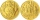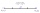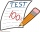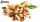# Equation - 7th grade (12y) - math problems - page 24

1. A numberA number increased by 7.9 is 8.3
2. MG=7x-15,MG=7x-15, FG=33, x=? Point M is the midpoint of FG. Find unknown x.
3. WhichWhich decimals when subtracted equal 3.89: a - b = 3.89
4. The quotientThe quotient of g and 55 is the same as 279. What is g?
5. Find unknownFind unknown numerator: 4/8 + _/8 = 1
6. GivenGiven 2x =0.125 find the value of x
7. EqnSolve equation with fractions: 2x/3-50=40+x/4
8. 1.5 divided1.5 divided by 1 = w divided by 4
9. Mother and daughterMother is 44 years old, her daughter 14. How many years ago was her mother four times older than her daughter?
10. Wood dividingWhich equation calculates the number of 1/3-foot pieces that can be cut from a piece of wood that is 7 feet long?
11. Midpoint 5FM=3x-4, MG=5x-26, FG=? Point M is the midpoint of FG. Use the given information to find the missing measure or value.
12. Midpoint 6FM=8a+1, FG=42, a=? Point M is the midpoint of FG. Find unknown a.
13. Far countryIn a country far away, the value of 3 pesos is 12 centavos more than the value of 1 peso. How many centavos is 1 peso worth?
14. Find midpointFM=5y+13, MG=5-3y, FG=? M is the midpoint of FG. Use the given information to find the missing measure or value.
15. Examination 2In an examination, a teacher awards 2 marks for every correct answer and subtract a mark for every wrong answer. If there are 20 questions and a child scored 25 marks how many correct answer did the child get?
16. Missing numberBlank +1/6 =3/2 find the missing number
17. Bag of peanutsJoe eat 1/3 of a bag of peanuts, mark eat 1/4 of the remaining in the bag of peanuts, Alvin eat 1/2 of the remaining bag of peanuts, peter eat 10 peanuts, there are 71 peanuts left. Hon many peanuts were in the bags?
18. One thirdIf 3/5 is 360, how much is 1/3?
19. Eq-fracSolve the following equation with fractions: h + 1/3 =5/3
20. Fraction + eqSolve following simple equation with fractions: -5/6(8+5b) = 75 + 5/3b

Do you have an interesting mathematical word problem that you can't solve it? Enter it, and we can try to solve it.

To this e-mail address, we will reply solution; solved examples are also published here. Please enter the e-mail correctly and check whether you don't have a full mailbox.

Please do not submit problems from current active competitions such as Mathematical Olympiad, correspondence seminars etc...

Do you have a linear equation or system of equations and looking for its solution? Or do you have quadratic equation?